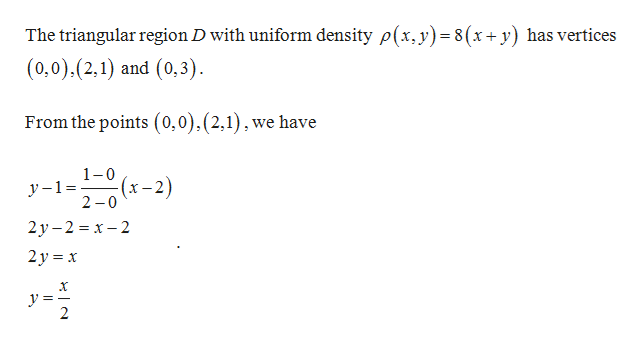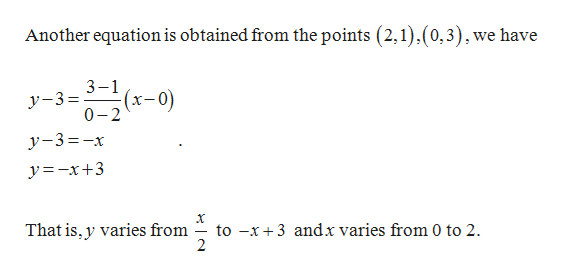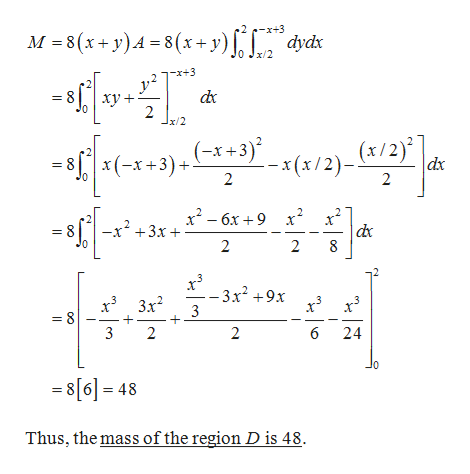# Find the mass and center of mass of the lamina that occupies the region D and has the given density function ρ.D is the triangular region with vertices (0, 0), (2, 1), (0, 3); ρ(x, y) = 8(x + y)

Question

Find the mass and center of mass of the lamina that occupies the region D and has the given density function ρ.

D is the triangular region with vertices (0, 0), (2, 1), (0, 3); ρ(x, y) = 8(x + y)
check_circleExpert Solution
Step 1

Given:help_outlineImage TranscriptioncloseThe triangular region D with uniform density p(x, y) 8 (x+ y) has vertices (0,0),(2,1) and (0,3) From the points (0,0).(2,1),we have 1-0 y-1 (x-. 2 0 2) 2у -2 %3Dх -2 2у %3D х X y=- 2 fullscreen
Step 2

Also,help_outlineImage TranscriptioncloseAnother equation is obtained from the points (2,1),(0,3),we have 3-1 (x-0) y-3= 0-2 (0-) y-3-x y=-x+3 to -x3 andx varies from 0 to 2 2 That is, y varies from fullscreen
Step 3

Find the mass M of the regi...help_outlineImage TranscriptioncloseM 8(x+y)A-8 (x+ y) dydx x/2 -x+3 2 * +3) *(x/2)_ (x/2)] x(-x+3) dx 2 2 x2-6x9x2 -x2 +3x 2 2 -3x2 +9x 3 3x2 = 8 3 2 2 6 24 0 8= 48 Thus, the mass of the region D is 48 fullscreen

### Want to see the full answer?

See Solution

#### Want to see this answer and more?

Solutions are written by subject experts who are available 24/7. Questions are typically answered within 1 hour*

See Solution
*Response times may vary by subject and question
Tagged in

### Math